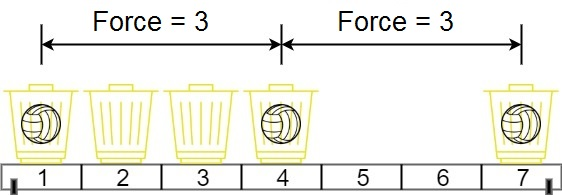1552. Magnetic Force Between Two Balls
Medium
1.8K
102

In the universe Earth C-137, Rick discovered a special form of magnetic force between two balls if they are put in his new invented basket. Rick has `n` empty baskets, the `ith` basket is at `position[i]`, Morty has `m` balls and needs to distribute the balls into the baskets such that the minimum magnetic force between any two balls is maximum.

Rick stated that magnetic force between two different balls at positions `x` and `y` is `|x - y|`.

Given the integer array `position` and the integer `m`. Return the required force.

Example 1:```Input: position = [1,2,3,4,7], m = 3
Output: 3
Explanation: Distributing the 3 balls into baskets 1, 4 and 7 will make the magnetic force between ball pairs [3, 3, 6]. The minimum magnetic force is 3. We cannot achieve a larger minimum magnetic force than 3.
```

Example 2:

```Input: position = [5,4,3,2,1,1000000000], m = 2
Output: 999999999
Explanation: We can use baskets 1 and 1000000000.
```

Constraints:

• `n == position.length`
• `2 <= n <= 105`
• `1 <= position[i] <= 109`
• All integers in `position` are distinct.
• `2 <= m <= position.length`
Accepted
42K
Submissions
71.8K
Acceptance Rate
58.5%

Seen this question in a real interview before?
1/4
Yes
No

Discussion (0)

Related Topics